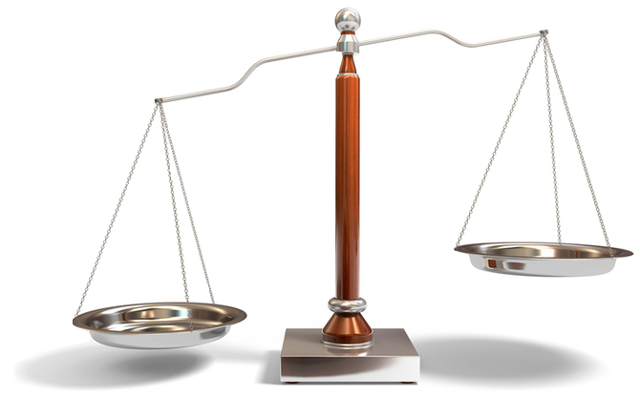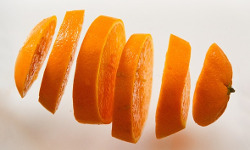# 3 ways to compare slices (arrays)

yourbasic.org/golang## Basic case

In most cases, you will want to write your own code to compare the elements of two slices.

``````// Equal tells whether a and b contain the same elements.
// A nil argument is equivalent to an empty slice.
func Equal(a, b []int) bool {
if len(a) != len(b) {
return false
}
for i, v := range a {
if v != b[i] {
return false
}
}
return true
}``````

For arrays, however, you can use the comparison operators `==` and `!=`.

``````a := int{1, 2}
b := int{1, 3}
fmt.Println(a == b) // false
``````

Array values are comparable if values of the array element type are comparable. Two array values are equal if their corresponding elements are equal. The Go Programming Language Specification: Comparison operators

## Optimized code for byte slices

To compare byte slices, use the optimized `bytes.Equal`. This function also treats nil arguments as equivalent to empty slices.

## General-purpose code for recursive comparison

For testing purposes, you may want to use `reflect.DeepEqual`. It compares two elements of any type recursively.

``````var a []int = nil
var b []int = make([]int, 0)
fmt.Println(reflect.DeepEqual(a, b)) // false``````

The performance of this function is much worse than for the code above, but it’s useful in test cases where simplicity and correctness are crucial. The semantics, however, are quite complicated.

### Further readingSlices and arrays in 6 easy steps